# Firming Up Hierarchy

If an unmarked package arrived at your door, how would you figure out what was inside? The catch is that you cannot open it.

As a social scientist, I deal with this ‘black-box’ problem all the time. I (metaphorically) watch people go to work at firms. And I see them come home with income. Then I try to picture the ‘machine’ that gave people money.

In my mind’s eye, I see a machine called hierarchy.

Inside each firm, I imagine a corporate hierarchy that sorts workers into a chain of command and assigns them income based on their rank. Or in more personal terms, when people go to work they have a boss. And their boss makes more money than them.

In a colloquial sense, we all know that this is how firms work, because we’ve experienced it as workers. In other words, as individuals, we get an ants-eye view of the corporate hierarchy. But what we can’t do is play god and study corporate hierarchy by cutting the ant’s nest in half. Corporations tend to dislike that.

And so as scientists, we’re left with a black-box problem. If we want to study how hierarchy affects income, we have to do it without opening the corporate box. Our only option is to ‘stand’ outside firms and watch what goes in and out. Then we see if these observations are consistent with what we think goes on inside.

Speaking of observations from firms, in this post, I unpack data from a landmark 2019 paper called ‘Firming up inequality’. In that article, economists Jae Song and colleagues use data from the Social Security Administration to reconstruct the income distribution within US firms from 1981 to 2013. Their results are a goldmine for studying the hierarchical pay structure within firms.

Using Song’s data, here’s the idea that I’m going to test. I think that the recent rise in US income inequality is being driven by a redistribution of income within firms. In short, I believe that corporate hierarchies have become more despotic. Corporate elites have taken income that once went to the bottom of the hierarchy and redirected it to the top.

To test this idea, we’ll take a meandering route. First, I’ll tell you about my model of corporate hierarchy and how it explains income as a function of ‘hierarchical power’. Then I’ll give you a tour of US income inequality, and show you why it’s plausible that the recent rise in top incomes is being driven by growing ‘hierarchical despotism’. Next, I’ll break out the math and build a model of the US corporate landscape. I’ll use this model to predict the redistribution of income within US firms. Finally, I’ll compare the model’s predictions to the real-world trends reported by Song and colleagues. If all goes well, we’ll get some insight into the machinations of US corporate hierarchy.

My results? I find that to a surprising extent, the redistribution of income within US firms can be explained by a single parameter — a change in the rate that income scales with hierarchical power.

### Modeling the corporate blackbox

If you’ve ever worked in a big corporation, you’ve probably noticed that it is hierarchically organized. When you go to work, you have a boss who tells you what to do. And your boss has a boss who tells them what to do. And so it on.

In general, this corporate chain of command can be quite complex. But for modeling purposes, lets suppose that it is simple. Imagine that within the corporate hierarchy, your boss has n direct subordinates. And your boss’s boss has n direct subordinates. And so it goes, all the way to the top of the hierarchy.

In this model (which was first proposed in the 1950s by Herbert Simon and Harold Lydall), we get simple hierarchies like the ones shown in Figure 1. Here, the shape of the hierarchy is determined by the number of subordinates controlled by each supervisor — a number we’ll call the span of control. If the span of control is large (as on the left) we get a ‘flat’ hierarchy. If the span of control is small (as on the right), we get a ‘steep’ hierarchy.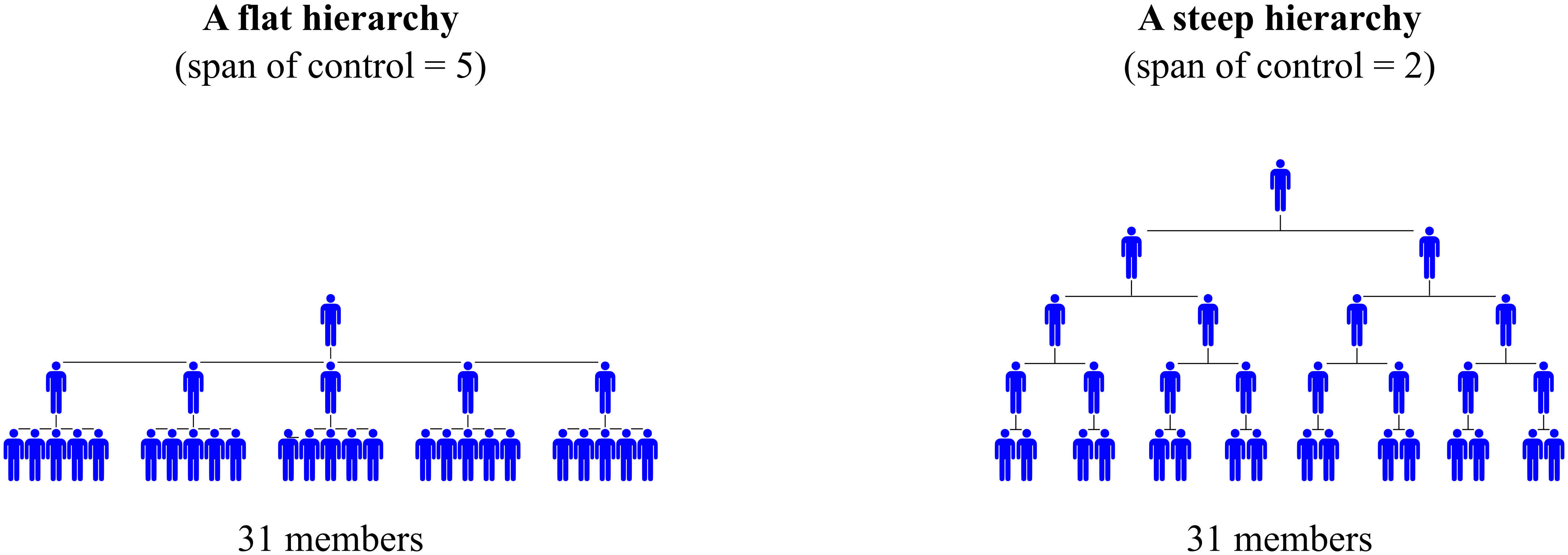Figure 1: A simple model of hierarchy. In my model of corporate hierarchy, I assume that each supervisor controls the same number of subordinates — a number we’ll call the ‘span of control’. If the span of control is large (left), we get a hierarchy that is flat. If the span of control is small (right), we get a hierarchy that is steep.

With this model in hand, let’s look at a universal feature of hierarchical organization: as you advance in rank, you accumulate subordinates. Figure 2 shows an example. Starting at the bottom of the hierarchy (rank 1), we find people with no subordinates. Moving to the second rank, we find individuals with 2 subordinates. People in the third rank have 6 subordinates. Managers in the fourth rank have 14 subordinates. And the top-ranked individual has 30 subordinates.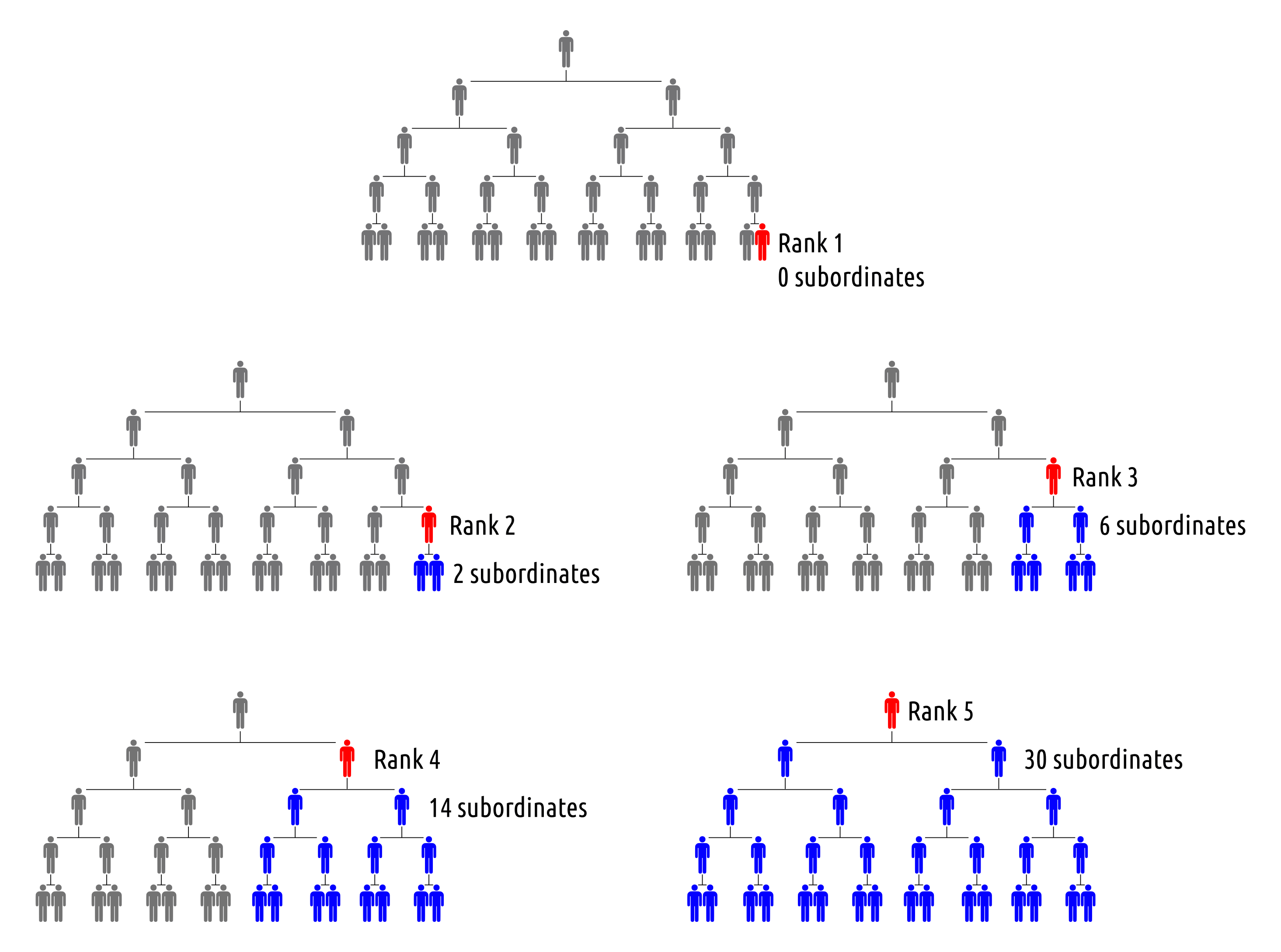Figure 2: As you move up the hierarchy, you accumulate subordinates. In each hierarchy, the blue individuals are subordinate to the red individual. As the red individual moves up the hierarchy, they accumulate subordinates.

Now, as you climb a real-world hierarchy, the exact way that you accumulate subordinates depends on the specifics of the chain of command. But in general, the number of subordinates grows exponentially with hierarchical rank. (In Figure 2, the pattern is n = 2^r - 2 , where n is the number of subordinates and r is hierarchical rank.)

Since the control of subordinates is a form of power, let’s call it that. Let’s define hierarchical power as:

\displaystyle \text{hierarchical power} = 1 + \text{number of subordinates}

The thinking here is that everyone starts with a baseline hierarchical power of one, indicating that they have control of themselves. From there, you gain hierarchical power by accumulating subordinates.

### The double-edged sword

Outside of economics, most social scientists accept that large human groups tend to be hierarchically organized, with a small elite holding the reigns of power. The controversy comes when we try understand what these elites do. Functionalists think that elites provide a service to society. Conflict theorists think elites are parasites who exploit their subordinates.

I think both schools of thought have merit.

In evolutionary terms, I view hierarchy as a group-level adaptation. When it functions well, the chain of command allows large groups to act cohesively. The ‘function’ of hierarchy, then, is to allow these groups to out-compete their neighbors. The problem is that this ‘function’ is achieved by concentrating power in the hands of the few. Inevitably, elites use this power for their own benefit. And so hierarchy becomes a double-edged sword. It is a tool both for group organization and for elite despotism. (I explore this duality in more detail here.)

Importantly, we see this hierarchical despotism on display everywhere. If elites used their power purely for the ‘good of the group’, they would share resources equally with their subordinates. And so we would find large hierarchies in which the rulers received the same income as bottom-ranked individuals. Such hierarchies do not exist.1 Instead, the norm is that income grows with hierarchical power.

Figure 3 illustrates this norm using data from a variety of institutions. Here, the horizontal axis shows individuals’ hierarchical power within their institution. The vertical axis shows their relative income within the hierarchy. The red dots show data from a handful of firm case studies. Blue dots show income and hierarchical power within the US military. And green dots show the income and hierarchical power of US CEOs. The black line illustrates the overall trend, which we can model nicely with a straight line. Income tends to grow with hierarchical power.Figure 3: Within hierarchies, income tends to increase with hierarchical power. This figure illustrates how income scales with hierarchical power within a variety of institutions. Red dots show data from a handful of firm case studies. Blue dots show data from the US military. Green dots show data for US CEOS. In the case-study firms and the US military, I measure income relative to the average in the bottom hierarchical rank. Each point indicates the average hierarchical power within a rank. For CEOs, I measure income relative to the average pay within the firm. I assume the CEO commands the firm, meaning their hierarchical power is equivalent to the firm’s total employment. For data sources, see the appendix in ‘Redistributing income through hierarchy’.

From the pattern in Figure 3, we can write a simple equation which describes income within a hierarchy. Income is proportional to hierarchical power, raised to some exponent D:

\displaystyle \text{relative income} \propto (\text{hierarchical power}) ^D

The parameter D determines how rapidly income grows with hierarchical power. If D is small, income grows slowly. For example, when D is 0.1, a CEO with one million subordinates would earn about 4 times more than an entry-level employee. But if D is 1, the same CEO would earn 1 million times more than a bottom-ranked employee. Figure 4 illustrates how the income pattern changes for different values of D.Figure 4: The degree of hierarchical despotism. Grey points show the empirical relation between income and hierarchical power (from Figure 3). Colored lines illustrate how income scales with hierarchical power for different values for D — the degree of hierarchical despotism. [Sources and methods]

In mathematical terms, the parameter D is just a slope — it is the rate that income scales with hierarchical power. But in social terms, D has a more incendiary meaning. It indicates the degree to which elites use their power to enrich themselves. For that reason, I call D the ‘degree of hierarchical despotism’.

### Becoming more despotic

Now we’re getting to the crux of how I understand hierarchy. It is a tool for both group organization and for elite despotism. Importantly, it seems likely that this despotism can vary in different ways. From Figure 4, we can tell that hierarchical despotism varies between institutions. It also likely varies between societies. And since culture can change, hierarchical despotism probably varies over time, both within institutions and within societies.

The big-picture goal of my hierarchy research is to understand how and why these despotism changes occur. The first step, however, is to simply establish that changes in despotism do occur. The idea is to see if there is a single parameter — the degree of despotism — that describes how income gets distributed (and redistributed) within hierarchies.

If firms were open boxes, this task would be simple. We’d look inside the corporate hierarchy and see if the model I’ve described is correct. But since firms are not open boxes (information about the chain of command is proprietary), we must use an indirect approach. First, we look for income data that plausible relates to corporate hierarchy. Then we see if our hierarchy model can predict this data.

With this goal in mind, here is the road ahead. After a brief tutorial on visualizing income redistribution, I’m going to build a model of hierarchy that replicates the redistribution of top incomes in the United States. From this model, I’ll infer how hierarchical despotism has changed within US firms. Then I’ll test this inference by looking at data from Song and colleagues, who report a trove of data for real-world US firms.

Yes, the test is a bit of a Rube-Goldberg machine. But it’s the kind of technique we must use when we study a black box. If all goes well, when we’re finished, we’ll know more about hierarchical despotism within US firms.

### The percentile view of income

We’ll start our foray into US income distribution with a brief tutorial on data visualization. I’m going to describe what I call the ‘percentile view of income’. We’ll use this tool to visualize how US income has changed over time.

To illustrate the percentile view, it’s helpful to start with data that’s more concrete than income. To that end Figure 5 shows the percentile view of American weight.

Here’s how the chart works. First, we take a sample of Americans and rank their weight from lightest to heaviest. Then we plot this rank as a percentile on the horizontal axis. (The lightest person is percentile 0 and the heaviest person is percentile 100.) On the vertical axis, we then plot each person’s actual weight. The resulting curve has a sideways Z-shape that highlights the extremes of weight. (The lightest 1% of Americans are under 100 pounds. The heaviest 1% are over 300 pounds.)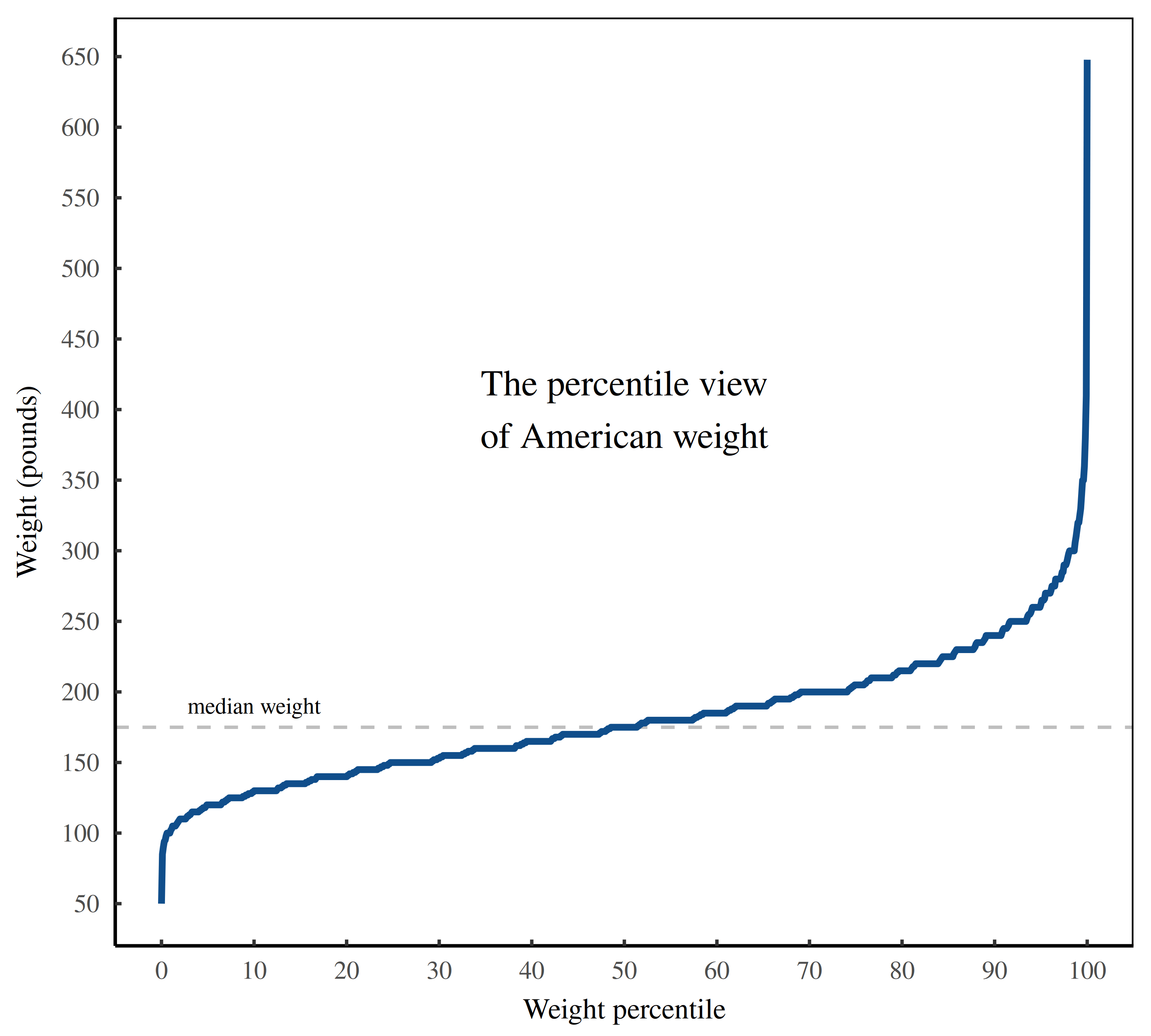Figure 5: The percentile view of American weight. This figure illustrates the weight distribution of a sample of US adults. The horizontal axis ranks individual weight on a percentile scale. The vertical axis plots the corresponding weight of each individual. The resulting Z-shape highlights the low and high extremes of weight. (The ‘steps’ in the data occur because weight is typically reported to the nearest 5 pounds.) Data is from the CDC.

Now that we understand the percentile view of weight, let’s look at the percentile view of income. Figure 6 shows the US distribution of income in 1981. Again, we see a Z-shape curve that highlights the extremes of low and high income. On that front, notice that the vertical axis uses a logarithmic scale: each tick mark corresponds to a factor of 10. We need this log scale to capture the true variation in income, which is enormous. In 1981, the richest Americans earned more than $10 million per year. The poorest Americans earned a few hundred dollars.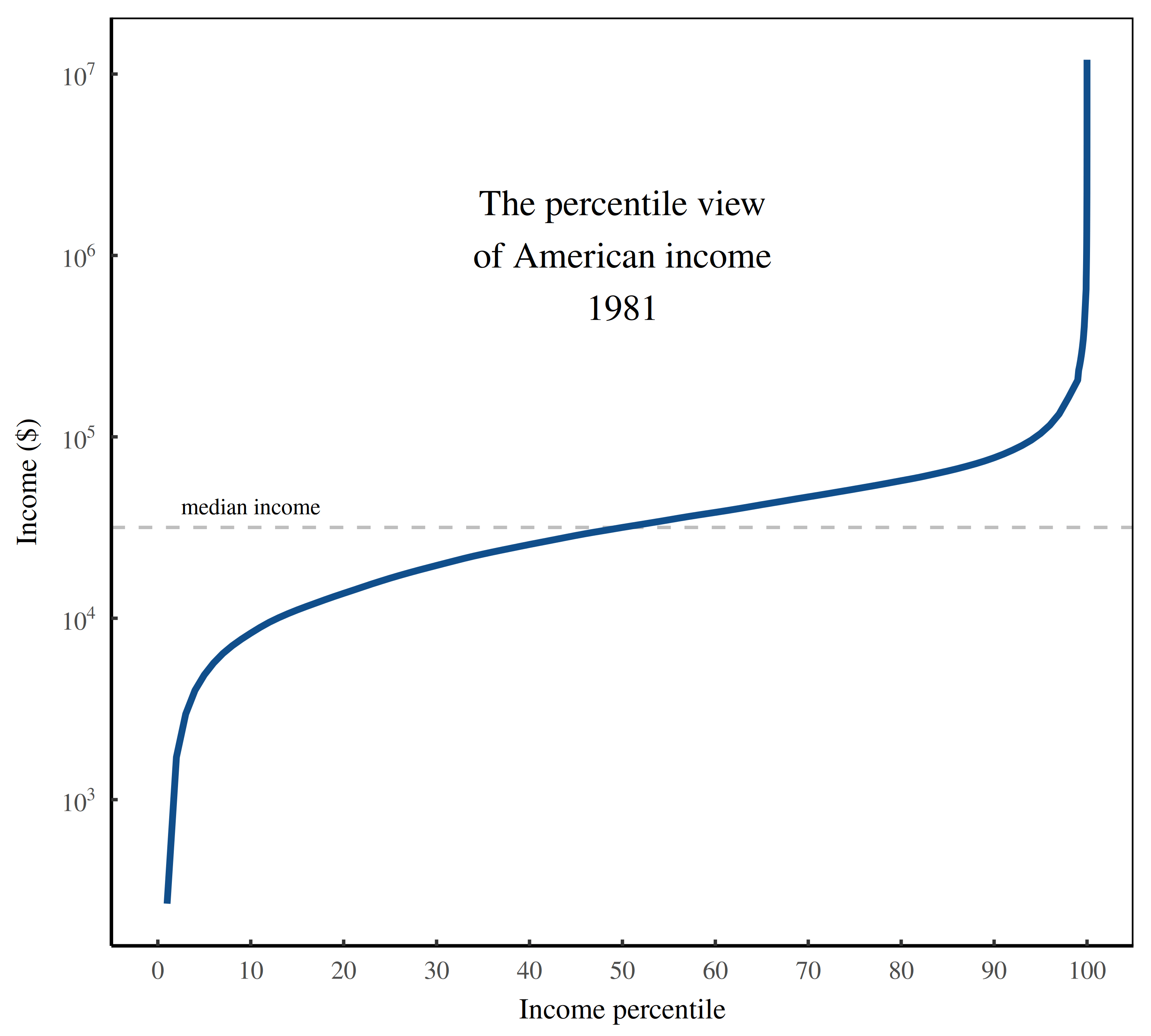Figure 6: The percentile view of US income in 1981. This figure illustrates the distribution of US income in 1981. The horizontal axis ranks individual income on a percentile scale. The vertical axis shows the dollar value of individual income, plotted on a logarithmic scale. [Sources and methods] Next, we’ll use the percentile view to see how the distribution of US income changed over time. But first, some prerequisites. As a rule, when we talk about the distribution of income, we care about relative income. Last year, for example, you may have earned$50,000. But we don’t care about this dollar value. What interests us is the size of your income relative to other people. Compared to Bill Gates, you are poor. Compared to a pauper, you are rich.

Here, I’ll measure income relative to the median. So by definition, the median income is 1, and everyone else’s income is measure relative to that value.

With relative income in mind, we’re ready to visualize the redistribution of US income. In Figure 7, I’ve plotted two snapshots of US income — one in 1981 (blue) and one in 2013 (red). As before, the horizontal axis ranks income on a percentile scale. But now, the vertical axis plots the relative size of income, measured against the median.Figure 7: The percentile view of US income in 1981 and 2013. This figure illustrates how the distribution of US income changed between 1981 (blue) and 2013 (red). The horizontal axis ranks individual income from lowest to highest, expressed as a percentile. The vertical axis shows the size of this income, measured relative to the median (in each year). The fact that the income curves do not overlap indicates that income has been redistributed. [Sources and methods]

Looking at Figure 7, we can see that the two income curves don’t overlap. That tells us that income has been redistributed. Between 1981 and 2013, the American poor got poorer. And the American rich got richer.

To get a clearer picture of this redistribution, let’s make one more tweak to our visualization. Instead of plotting the relative size of income on the vertical axis, let’s plot the change in this relative size. For example, in 1981, individuals at the 99th percentile earned 6.5 times the median income. But in 2013, the same percentile earned 10.4 times the median income. So these 99 percenters saw their (relative) income grow by about 60%. To visualize how US income got redistributed between 1981 and 2013, we’ll repeat this calculation for every income percentile.

Figure 8 shows the results. Here, income percentile is again on the horizontal axis. But now the vertical axis shows the change in relative income between 1981 and 2013. (By definition, the median income remains unchanged.) We see that most of the redistribution action happened among top incomes. The richest Americans saw their relative income grow by a factor of 5.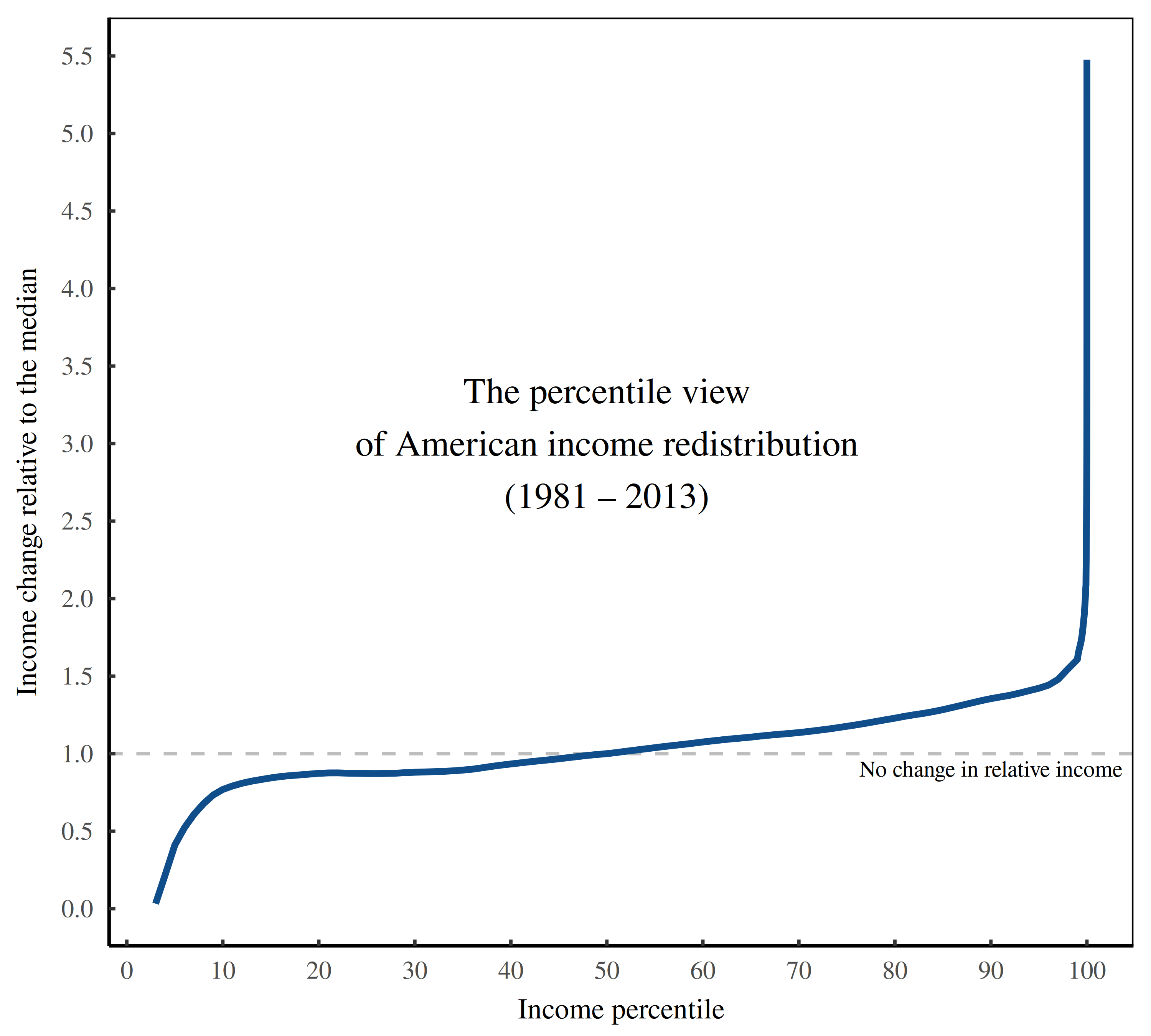Figure 8: The percentile view of US income redistribution, 1981 to 2013. This figure shows how US incomes changed (in relative terms) between 1981 and 2013. The horizontal axis ranks individual on a percentile scale. The vertical axis shows the change in income, measured relative to the median (in each year). Most of the redistribution action happened among the rich. [Sources and methods]

Now we’re getting to the core of the redistribution puzzle. Over the last four decades, a mysterious social change caused top US incomes to explode, but left the majority of incomes largely unchanged (in relative terms). The result is an L-shaped redistribution of income, shown in Figure 8.

What caused this transformation? I think the answer is simple: US firms became more despotic.

### Some key features of hierarchy

To build the case that US firms have become more despotic, we’ll start by reviewing some key features of hierarchy. To that end, let’s look at Figure 9.

Here, I’ve visualized a hierarchy that contains 100,000 members, organized with a span of control of 3. (In other words, each superior controls three subordinates.) As we move up the hierarchy, membership in each consecutive rank declines exponentially. The result is a bottom-heavy pyramid, pictured in Figure 9A.

Because hierarchy concentrates membership at the bottom, the corollary is that it concentrates power at the top. So when we look at individuals’ hierarchical power, we get a pyramid that is top heavy. Figure 9B illustrates. As we move up the hierarchy, hierarchical power increases exponentially. (Remember that I define hierarchical power as the number of subordinates + 1.)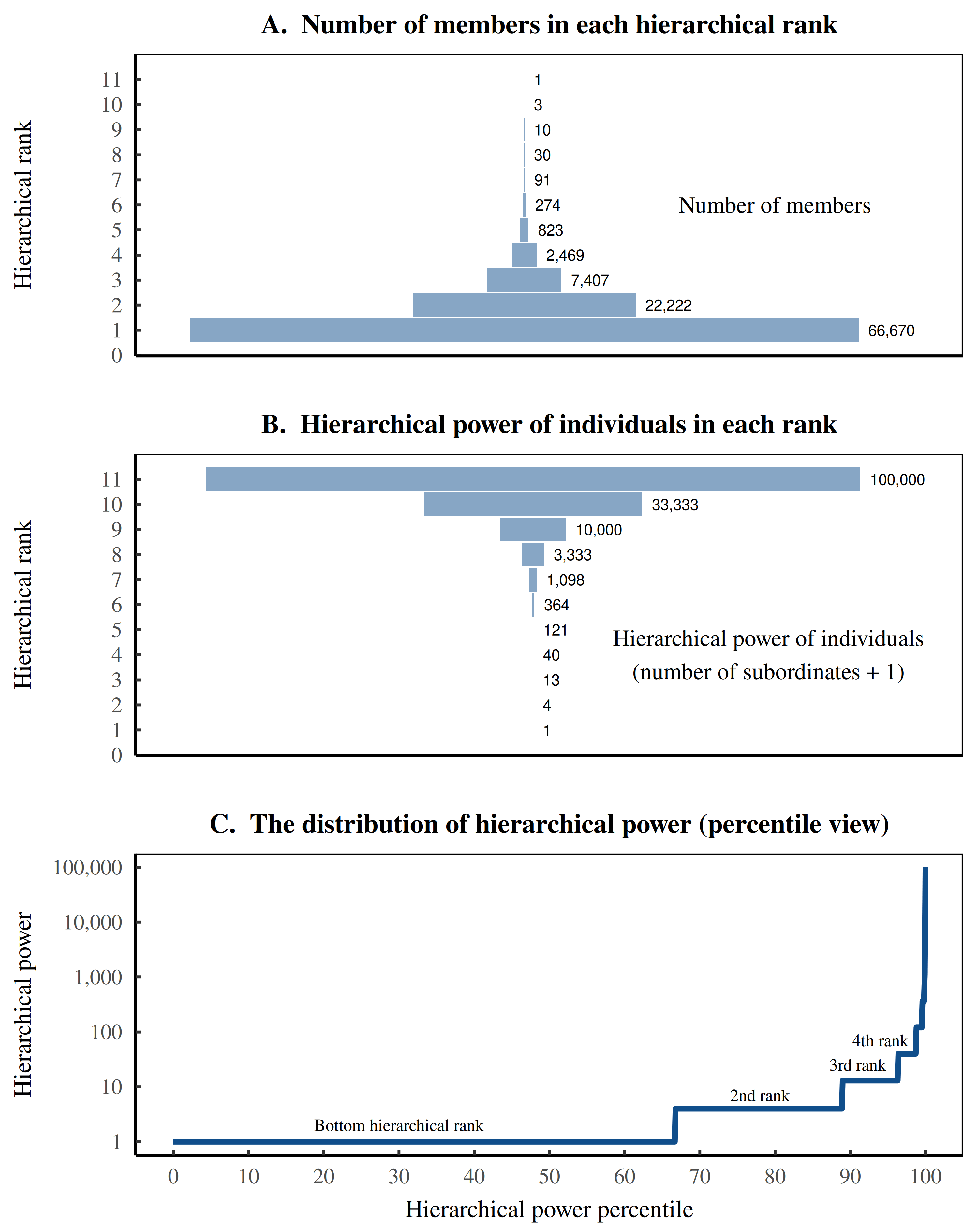Figure 9: Hierarchy creates bottom-heavy membership, top-heavy power, and an L-shaped percentile view of power. This figure illustrates the features of a modeled hierarchy with 100,000 members, organized with a span of control of 3. Panel A shows the number members in each rank — a bottom-heavy pyramid. Panel B shows the hierarchical power of individuals in each rank — a top-heavy pyramid. (Hierarchical power is defined as the number of subordinates + 1.) Panel C shows the distribution of hierarchical power within the hierarchy, visualized using the percentile view. The horizontal axis ranks the hierarchical power of individuals from lowest to highest. The vertical axis shows individuals’ corresponding hierarchical power, plotted on a log scale. The ‘steps’ in the curve correspond to jumps in hierarchical rank. [Sources and methods]

Now, just as we did with income, we can take our hierarchy and visualize its distribution of hierarchical power using the percentile view. When we look at this distribution of power, we are combining two features of hierarchy — the fact that (a) membership is bottom heavy and (b) power is top heavy. The consequence of these opposing features is that within a hierarchy, the vast majority of people have virtually no power, while a tiny elite wields enormous control.

When we plot the resulting distribution of power using the percentile view, we get an L-shaped pattern, as shown in Figure 9C. Some things to note. Since hierarchical power varies over an enormous range, I’ve used a log scale on the vertical axis. So each tick mark indicates a factor of 10. Also notice the step-like pattern in the distribution of hierarchical power. Each ‘step’ corresponds to a jump in hierarchical rank, a jump that brings with it more subordinates, and hence, more hierarchical power.2

### Distribution through despotism

To review, hierarchy gives rise to an L-shaped distribution of power. If we assume that income scales with hierarchical power, then we automatically get an L-shaped distribution of income. Figure 10 illustrates.

Here, I’ve taken the hierarchy from Figure 9 and added a scaling relation between hierarchical power and income — a relation that I call the ‘degree of despotism’. Then I’ve plotted the resulting distribution of income using the percentile view. The colored curves show the pattern for various degrees of despotism (the rate that income scales with hierarchical power). The result is an L-shaped curve that can be scaled up or down by varying the degree of despotism.3Figure 10: Distribution through despotism. This figure illustrates the distribution of income within a hierarchy, assuming that income scales with hierarchical power. I start with the hierarchy shown in Figure 9. Then I model income by assuming it scales with hierarchical power. I call the scaling rate the ‘degree of despotism’. When we plot the resulting distribution of income using the percentile view, we get an L-shaped curve. The colored lines show the pattern for various degrees of despotism. As we increase despotism, we scale up the L, preferentially increasing top incomes. [Sources and methods]

To summarize, if income scales with hierarchical power, we get an L-shaped distribution of income within hierarchies. Next question: what happens if we vary this scaling relation, meaning we change the degree of despotism? In that case, we get an L-shaped redistribution of income. Figure 11 illustrates.

Here I’ve taken the hierarchy from Figure 9 and imagined that over time, it grows more despotic. The hierarchy starts with despotism equal to 0.1 (meaning income scales with hierarchical power, raised to the exponent 0.1). At some later time, the hierarchy ends with despotism equal to 0.4. In Figure 11, the blue curve shows the resulting redistribution of income, plotted using the percentile view. Again, we get an L-shaped pattern. Ramping up despotism preferentially increases top incomes.Figure 11: Redistribution through despotism. This figure illustrates how ramping up hierarchical despotism redistributes income within a hierarchy. Using the hierarchy visualized in Figure 9, I assume it starts with a degree of despotism D = 0.1. Over time, I imagine that the hierarchy grows more despotic, ending with despotism D = 0.4. The blue curve shows how income gets redistributed as a function of income percentile. (Note that the vertical axis plots the change in income relative to income in the bottom hierarchical rank.) [Sources and methods]

At this point, you probably see where I’m headed. Within a hierarchy, ramping up hierarchical despotism produces an L-shaped redistribution of income (on the percentile view). And over the last forty years, the United States has experienced an L-shaped redistribution of income (Figure 8). It’s not exactly rocket science to think that the two L’s are connected.

What is difficult, though, is actually investigating the connection. As I’ve stressed repeatedly, we cannot simply open up US firms and see if their hierarchies have become more despotic. For the most part, the relevant data doesn’t exist. And so we have to make do with an indirect approach. To that end, the first task is to create a more realistic model of hierarchy — one that simulates not just a single firm, but the whole US corporate landscape.

### Modeling the US corporate landscape

So far, we’ve modeled at how despotism affects income within a single hierarchy. Doing so makes the math easy, but is not particularly realistic. In the real-world United States, there are millions of different corporate hierarchies (i.e. firms). So if we want our model to mimic the real world, we should simulate this corporate landscape.

The task sounds difficult, but is actually straightforward … provided we make some simplifying assumptions. Let’s start with the size distribution of firms. To a first approximation, this distribution follows a power law. In the United States, the probability of finding a firm with n employees is roughly proportional to 1/n^2 . In other words, compared to firms with a single member, there are 100 times fewer firms with 10 employees. And there are 10,000 times fewer firms with 100 employees. And so on.

Although this pattern deserves an explanation, for the moment, let’s take it as a given. The power-law pattern means it is fairly easy to simulate the US corporate landscape. We simply draw random numbers from a power-law distribution. Each number then represents the employment within an individual firm.

Once we’ve got a firm, we need to simulate its hierarchical structure. In principle, this simulation could be quite sophisticated, allowing for a different chain of command within each firm. But since we know virtually nothing about this complexity, let’s ignore it. Instead, let’s assume that every firm has the same simple structure, described by a single span of control. For example, if the span of control is 2, every superior in every firm has exactly 2 subordinates.

Given this assumption, we can take each firm (sampled from our power-law distribution) and endow it with a hierarchical structure. The math is straightforward, but not something we’d want to do by hand for millions of firms. Fortunately, a computer can crunch the numbers in a few milliseconds. While we’re at it, we can ask the computer to take each hierarchy and calculate each member’s hierarchical power. Once we’ve got this power, we can then calculate income. We assume that everyone’s income is proportional to their hierarchical power, raised to some exponent D (which I call the degree of hierarchical despotism).

At this point, income is exactly proportional to hierarchical power — something that’s not realistic. (It’s as if income was determined by natural law.) To make the model less naive, we’ll add some statistical noise to people’s income. So instead of a strict relation between hierarchical power and income, we have a strong trend.

The end result looks something like Figure 12. Here, I’ve take an iteration4 of the US hierarchy model and visualized it as a landscape. Each pyramid represents a firm, with size indicating the number of employees. You can see that most firms are small, but a few are extremely large — a characteristic feature of the power-law distribution of firms. Moving up each pyramid corresponds to moving up the corporate hierarchy. A we climb the hierarchy, income grows exponentially, as indicated by color.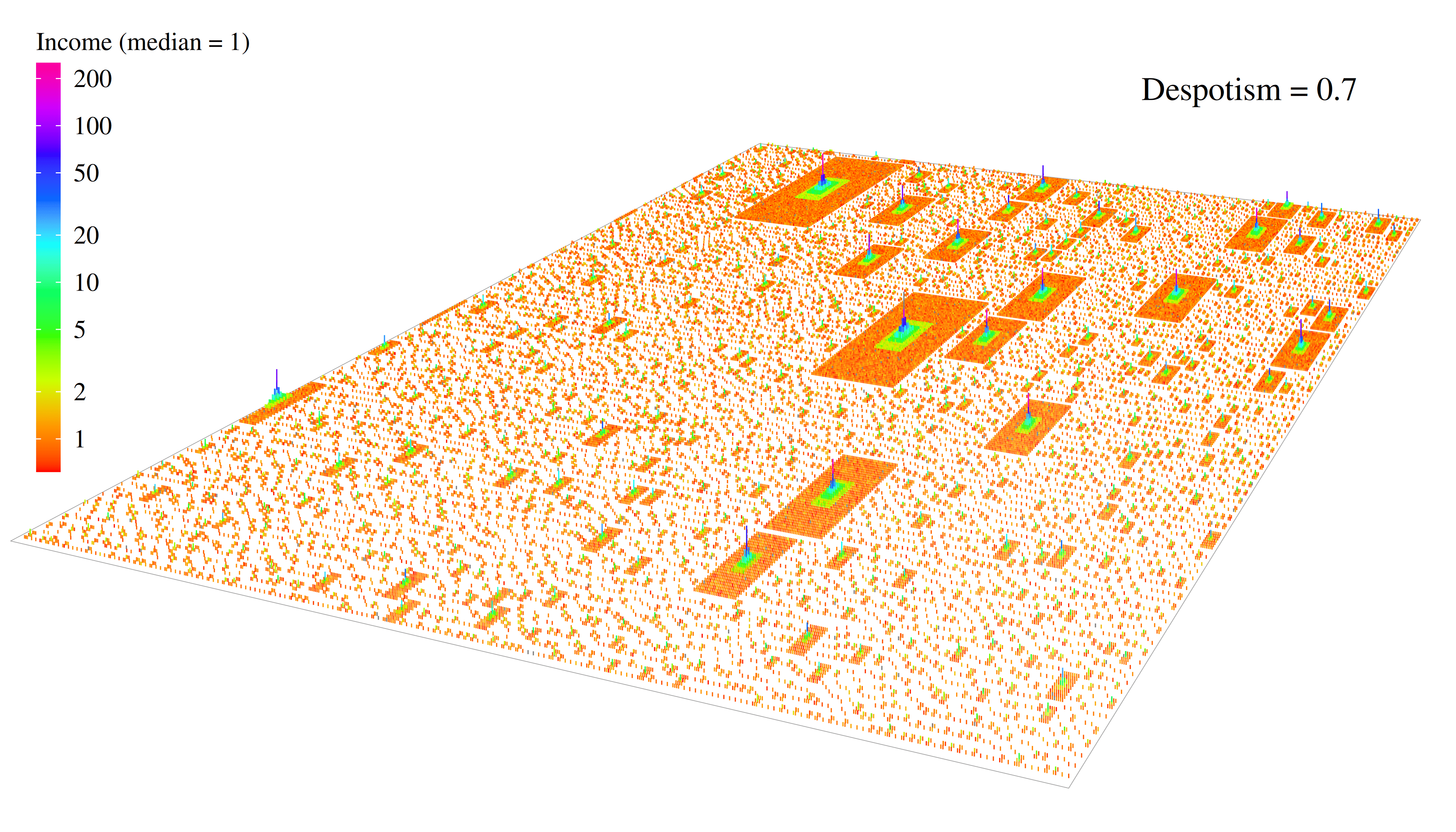Figure 12: Visualizing the US hierarchy model. This figure visualizes the US hierarchy model as a landscape. Each pyramid represents a firm. Moving up the pyramid corresponds to moving up the hierarchy. I’ve plotted individual income using color. [Sources and methods]

### Tuning in despotism

Now that I’ve outlined the features of my hierarchy model, it’s time to get philosophical. All models have parameters that can be ‘tuned’, meaning we can adjust them to fit observations. What’s important is that tuning the model is not the same thing as testing it. To test a model, you tune the parameters on one set of data. Then you test the model’s predictions on a different set of data. (Alternatively, you can tune the model’s parameters on two different sets of data and see if you get similar results.)

Here’s how I tune the US hierarchy model. I start with the size distribution of US firms, which I fit with a power-law distribution. This distribution then determines the sizes of firms in the model. Next, I look at case studies of institutional hierarchy and measure the average span of control. I use this average in the hierarchy model. Then, within these case-study institutions, I measure the noise in the power-income relation, and use that noise in the hierarchy model.

With these three parameters pinned down, that leaves one unknown — the degree of hierarchical despotism (the rate that income scales with hierarchical power). To set this parameter, I tune the hierarchy model to reproduce the distribution of US top incomes. (For details about how I tune the hierarchy model, see the Sources and methods.)

Form the hierarchy model, I infer that since the late 1970s, US firms have become steadily more despotic. Figure 13 illustrates. Here, the vertical axis shows the degree of US hierarchical despotism, inferred from the hierarchy model. As expected, we find that over the last four decades, despotism has increased.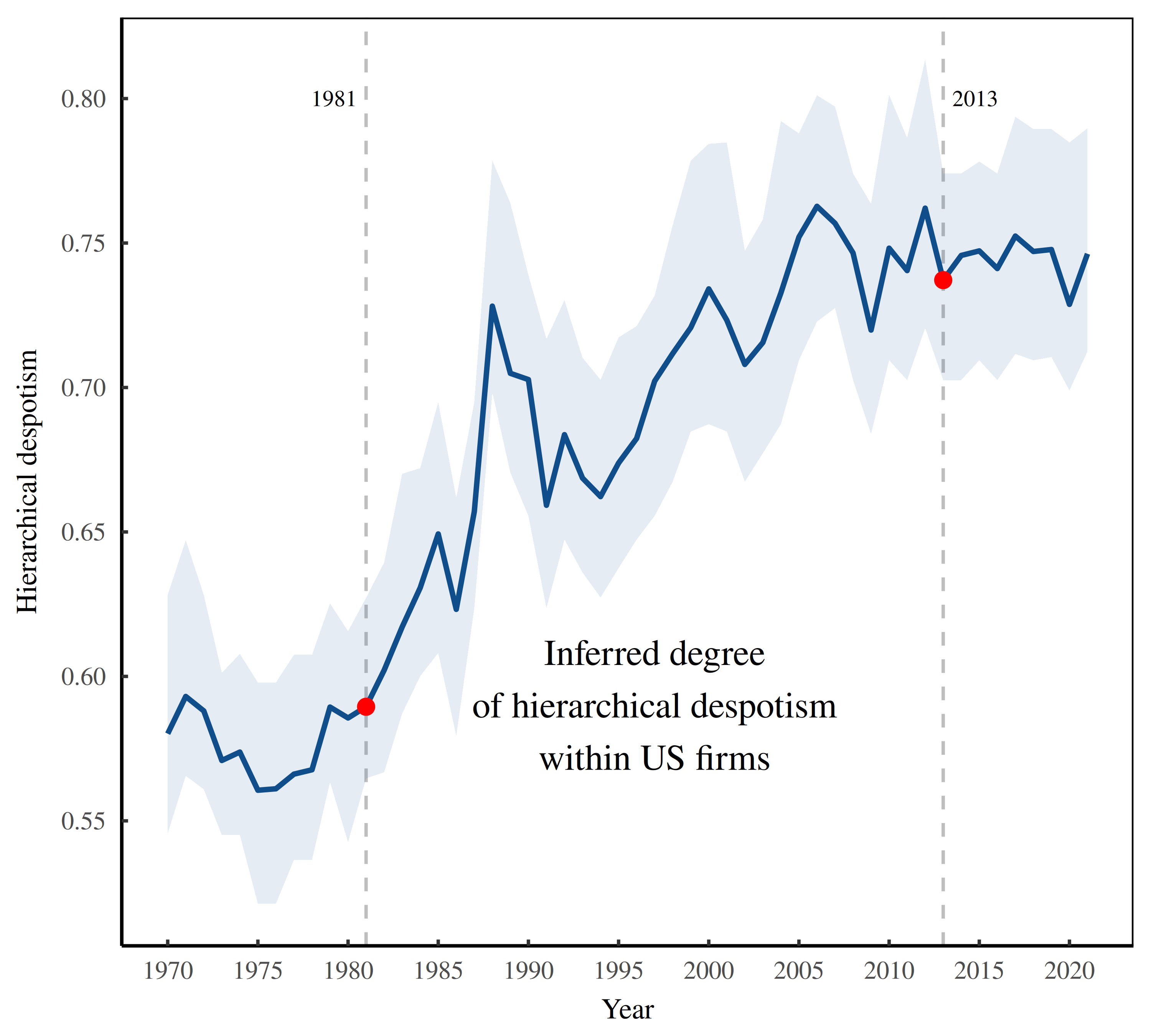Figure 13: Hierarchical despotism within US firms, inferred from the hierarchy model. This figure illustrates inferred values for the (average) degree of despotism within US firms. I estimate these values by fitting the hierarchy model to the US distribution of top incomes. Since the hierarchy model is stochastic, it returns a range of best-fit values, shown by the blue shaded region. The blue line shows the median value. Looking ahead, the red points in 1981 and 2013 show the despotism values I use to model the within-firm income data from Song and colleagues. [Sources and methods]

Now, I say that I ‘expected’ that US despotism would increase because this inference is (nearly) a foregone conclusion.5 The hierarchy model assumes that changes in inequality are driven by changes in hierarchical despotism. So if inequality increases (as it has in the US), the hierarchy model will infer that firms have become more despotic.

What’s interesting is not the inference per se, but whether this inference is correct. To that end, have a look at the red points in Figure 13. These are my inferred values for US hierarchical despotism in 1981 and 2013. These dates are significant because they represent the starting point and ending point of Song and colleague’s study of inequality within US firms. Their data offers a rare opportunity to test the despotism thesis.

### Firming up inequality

In a 2019 paper called ’Firming up inequality, Jae Song and colleagues used data from the Social Security Administration to reconstruct the distribution of income within (and between) US firms. Their source data comes from the SSA ‘Master Earnings File’, which is accessible only to Social Security employees (like Song). However, their paper and accompanying supplementary material make available a trove of data about the dynamics of income within US firms. I’m going to use this data to test the despotism thesis.

My test will be indirect in the sense that Song’s data does not explicitly measure the hierarchical structure within firms. That’s for us to infer. To that end, I’m going to compare predictions from the US hierarchy model to firm-level data from Song. From there, we’ll pass judgment on the despotism thesis.

### Counterfactuals

Song and colleagues use a counterfactual thought experiment to measure the redistribution of income within US firms. Since this thought experiment is not particularly intuitive, it’s worth breaking down the thinking behind it.

When we study the redistribution of income, we are generally interested in changes in relative income. (For example, we don’t care that you got a $10,000 raise. What interests us is the size of this raise relative to your neighbors.) When we think in these relative terms, we are actually thinking in counterfactuals. We imagine an alternative world in which one person’s income stays constant. Then we measure how everyone else’s income changes relative to that person. Of course, we rarely choose an actual person. Instead, we choose a statistical person, such as the individual with the mean (average) income. In essence, we’re imagining a counterfactual world in which the mean income stays constant. When we study income redistribution within firms, we can apply the same principle. The difference is that instead of holding one person’s income constant, we choose someone within each firm and fix their income. You’re probably familiar with this kind of counterfactual statistic. It’s the reasoning behind the CEO pay ratio — the ratio of CEO pay relative to the income of an average worker within the firm. When we study how this ratio changes with time, we are imagining a world in which the firm’s average pay stays constant. Then we judge how the CEO’s (relative) pay has changed. What Song and colleagues do is take this principle and apply it to everyone within the firm. They imagine a counterfactual United States in which the average income within all firms remains constant. (To calculate the average income, Song and colleagues use the geometric mean rather than the arithmetic mean.) Then they measure how incomes changed around this average. From this calculation, Song reports a plethora of fine-grain data on the income of individuals. Here I’ll focus on the percentile view of income redistribution — the picture of how income has changed as a function of percentile rank. When interpreting the data, keep in mind that it is counterfactual — what we would observe if the average income within all firms remained constant, but income redistribution within firms went ahead as it did historically. ### L-shaped redistribution within firms The picture that emerges from Song’s data is that within US firms, income redistribution has an L-shape. Figure 14 shows the trend. Let’s break down what you’re looking at. On the horizontal axis, I’ve plotted income percentile. The catch is that this is a counterfactual percentile. As before, I’ve ranked everyone’s income from smallest to largest. But this ranking is done on income measured relative to the average within each person’s respective firm. Given this counterfactual income percentile, the vertical axis shows the relative change in income between 1981 and 2013. The blue curve shows the US trend estimated by Song and colleagues. The red curves shows the trend predicted by the US hierarchy model.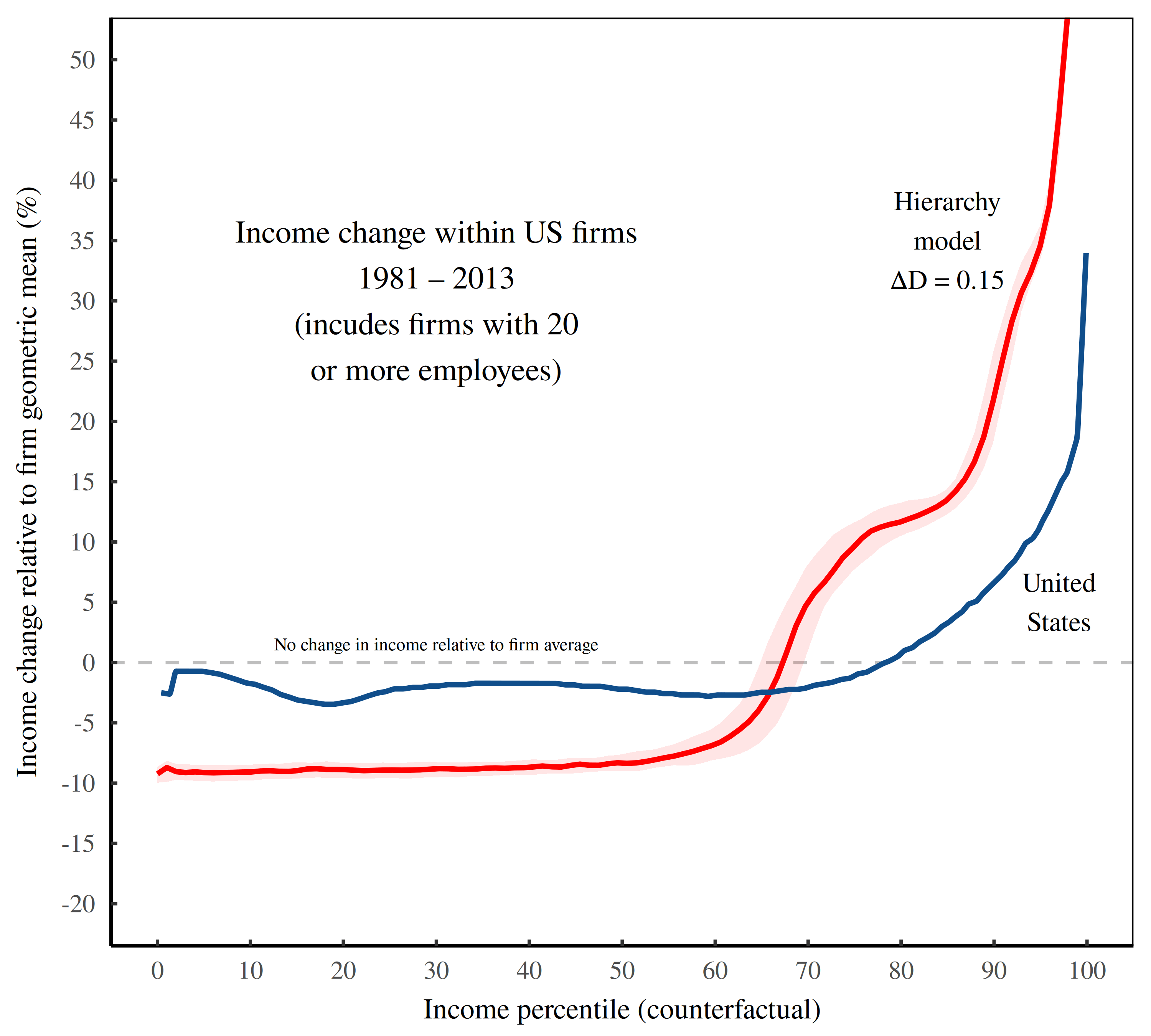Figure 14: Income redistribution within US firms. This figures shows the results of a counterfactual thought experiment in which we hold constant the average income within each US firm (measured using the geometric mean). We then rank each person’s relative income from smallest to largest and plot it as a percentile on the horizontal axis. On the vertical axis we measure the change in the size of this relative income between 1981 and 2013. The blue curve shows US estimates from Song et al. The red curves shows the US hierarchy model’s prediction. The red shade region shows the model’s range over many iterations. Notice the bumps in the model’s prediction? Those are caused by jumps in hierarchical rank. [Sources and methods] Now, it’s obvious that the hierarchy model’s prediction is not quite right. But before we get to the differences, let’s talk about the similarities between the real-world US and the model. In both cases, the redistribution pattern follows an L-shape. The question is, why? In the hierarchy model, we know the answer. The horizontal part of the L is produced by the roughly 60% individuals who sit at the bottom of each firm’s hierarchy. When we ramp up despotism, their income doesn’t change.6 The result is that we get a flatline on the percentile view of income redistribution. It’s only once we leave the bottom rank that income starts to grow. In the hierarchy model, this change happens around the 60th percentile. In Song’s US data, the flatline starts to break around the 70th percentile. Why the difference? One possibility is that we’ve assumed a span of control that is not quite right. You see, the span of control affects how many people are in the bottom hierarchical rank. And that number, in turn, affects where the flatline (of no income change) breaks. Based on a sample of case-study data, I’ve assumed a span of about 3. If we bumped that value up a bit (putting more people in the bottom rank of each hierarchy), we’d better match Song’s data. Looking at Figure 14, we can also see that the hierarchy model overestimates income growth among the top third of people. We can ‘fix’ this overestimate by lowering the change in despotism from 1981 to 2013. However, doing so wrecks the results to come (the view among the top 1%). And so we are left with a puzzle. Our model makes a prediction that is close to being right, but not quite there. It’s one of the frustrating parts of modeling, I’m afraid. Yes, we can tweak parameters to fit the data. But then we’re ‘tuning’ the model, not testing it. Until more data comes along, we’re left making guesses about the source of the discrepancy. ### The view from the top Let’s switch gears now and zoom into the top 1% of incomes. Here, the US hierarchy model makes strikingly accurate predictions for how income has been redistributed within US firms. Figure 15 illustrates. This chart shows the same counterfactual scenario as in Figure 14. (It measures income redistribution between 1981 and 2013, supposing that all US firms had their average income held constant.) But instead of showing all income percentiles, I’ve zoomed into the top 1%. So in Figure 15, the horizontal axis shows sub-percentiles among the top 1%. And the vertical axis shows the change in income among this group. (For comparison purposes, I’ve re-indexed the income change so it starts at zero at the 99th percentile.) The hierarchy model (red) predicts a redistribution of income that’s surprisingly similar to what we find in the real-world US (blue).Figure 15: Income redistribution within US firms — the view from the top. As in Figure 14, this figure illustrates a counterfactual scenario in which the average income (measured using the geometric mean) remains constant within all US firms. The horizontal axis then ranks the resulting top 1% of incomes. The vertical axis shows the percentage change in income between 1981 and 2013, measured relative to the 99th percentile. Song’s data for the US is shown in blue. Predictions made by the US hierarchy model are plotted in red. The shaded region indicates the range of model results. [Sources and methods] It’s worth emphasizing what these results show. It seems that there is a direct connection between the redistribution of top incomes in the United States as a whole, and the redistribution of top incomes within US firms. If we assume that firms have become more despotic — shifting income from the bottom to the top of the hierarchy — then the pieces fall together. We can take a model fitted to macro-level income data and use it to predict the redistribution of income at the tops of US firms. ### Hierarchical despotism revealed? If you were to guess the contents of an unopened box, the game would be fun for a while. But eventually, you’d want to open the box to see if you were right. When we do science, the frustrating part is that often the box can never be opened. Instead, we’re forced to keep probing the thing from different angles, slowly accumulating more evidence. Fortunately, this doesn’t mean the box’s contents are a mystery forever. As we accumulate data, the various strands of evidence usually point in a single direction. When it comes to the despotism thesis, we’re in the early stages of this investigation. And in a sense, that’s odd. For decades, US income inequality has been rising. And the evidence that this inequality is being driven by corporate despotism is not exactly subtle. Relative to the average worker, CEO pay has skyrocketed. So you’d think that economists would be clamoring to test the despotism thesis. But in large part, they are not. The roadblock is mostly ideological. In the eyes of mainstream economists, firms are units of ‘production’. So if CEO pay increases, it’s because CEOs have become more ‘productive’. It couldn’t be because CEOs have used their power to enrich themselves. No, that (straightforward) explanation would ruin the ideology that mainstream economists are pushing — the ruse that all is fair in capitalism. The alternative that I’ve proposed here is that firms are not units of production; they are units of power.7 Firms are hierarchical organizations shrouded in the cloak of corporate law. And today’s corporate rulers — like all elites before them — use their power to enrich themselves. On that front, the Song evidence suggests that US elites have recently upped their game, sending a torrent of income from the bottom to the top of the corporate hierarchy. In short, US firms have become more despotic. Or at least, that’s my black-box hypothesis. Admittedly, we’re a long way from having many lines of evidence that support the despotism thesis. But hopefully this situation will change with time, as more social scientists start to connect income with hierarchy. And regarding the Song data, I’ve only scratched the surface. So stay tuned for another dive into US hierarchical despotism. #### Support this blog Economics from the Top Down is where I share my ideas for how to create a better economics. If you liked this post, consider becoming a patron. You’ll help me continue my research, and continue to share it with readers like you.#### Stay updated Sign up to get email updates from this blog.This work is licensed under a Creative Commons Attribution 4.0 License. You can use/share it anyway you want, provided you attribute it to me (Blair Fix) and link to Economics from the Top Down. [Cover image: generated with Flametree] ### Sources and methods #### Data from Song et al Data for within-firm income redistribution (shown in Figure 14) is from Song et al Figure IV. Top 1% data (Figure 15) is from Song’s appendix, Figure A.12. I extracted data from both figures using Engauge Digitizer. #### US income distribution Data for the US distribution of income comes from the World Inequality Database, series ptinc992j, which reports income thresholds for corresponding income percentiles. #### Size distribution of US firms To estimate the size distribution of US firms, I use the following data: • Table bds_fsz_release from the US Census, Business Dynamics Statistics (BDS) • BLS series LNU02032185, self-employed workers, unincorporated agriculture • BLS series LNU02032192, self-employed workers, unincorporated nonagriculture industries The BDS data reports annual firm counts in various firm-size bins. Importantly, this data excludes self-employed workers who run unincorporated businesses. I add these workers to the smallest firm size bin using the above BLS series (the sum of agriculture and non-agriculture self-employed workers who are unincorporated). Figure 16A shows the resulting size distribution of US firms. The horizontal axis plots firm size. The vertical axis shows the density of firms by size. (Points indicate the midpoint of firm-size bins. Firm ‘density’ is the ratio of relative firm counts divided by the bin width.) The blue line represents US data from 1977 to 2014. The red line shows the power-law distribution that best fits the empirical data.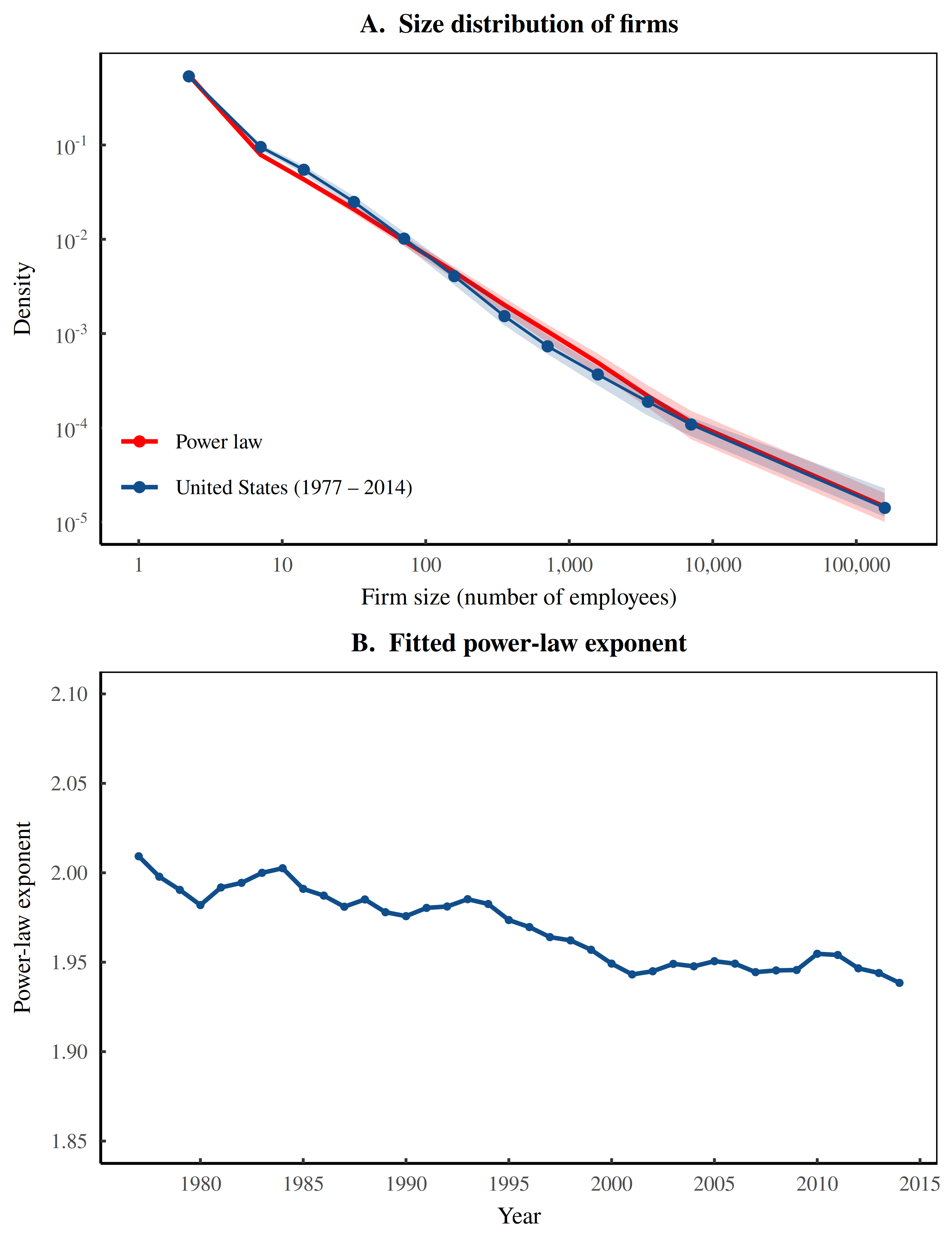Figure 16: Fitting the size distribution of US firms with a power-law distribution. This figure illustrates how the size distribution of US firms can be modeled with a power-law. Panel A shows how the relative number of firms varies with firm size. (Note the log scale on both axes.) The blue line represents US data from 1977 – 2014. The red line shows the best-fit power law. Shaded regions show the 99% range of data. Panel B shows how the best-fit power law exponent varies by year. This exponent, \alpha , means the probability of finding a firm of size x is roughly 1/x^{\alpha} . In Figure 16B, I fit a power law to annual firm-size data. The vertical axis shows the best-fit exponent as a function of time. As a reminder, the exponent \alpha describes the probability of finding a firm of size x : \displaystyle p(x) \propto \frac{1}{x^{\alpha}} The fitted values of \alpha average 1.97, but have trended downward over time (meaning the relative number of large firms is increasing slightly). To fit the power law, I use the binned maximum likelihood method outlined by Virkar and Clauset. In the fit, I exclude the first firm-size bin in the US data. Here’s why. Empirical data never follows a perfect power law, which means that when we fit a power law to it, the results are affected by the data we choose to emphasize. Including the first firm-size bin in the fit leads to a power-law that better captures the bottom of the distribution, but more poorly captures the relative number of large firms. Since it is these large firms that are most important for studying hierarchy, I deem it more important to fit the tail of the firm size distribution. Back to the hierarchy model. To capture uncertainty in the size distribution of firms, each iteration of the model samples randomly from the fitted power-law exponents shown in Figure 16B. #### Span of control Despite the ubiquity of hierarchical organization, there are few case studies of real-world hierarchies. Figure 17 illustrates the empirical data that I’ve been able to find. It includes: Panel A shows the distribution of the span of control within these institutions. Note that the span is defined as the ratio of aggregate employment in adjacent hierarchical ranks. (To my knowledge, there are no studies that resolve the chain of command down an individual-level network.)Figure 17: The span of control in case studies of hierarchy. This figure shows how the span of control varies among a handful of institutional case studies. The data includes six case studies of individual firms, as well as data from the US military. Panel A shows the distribution of the span of control within these institutions. Panel B shows the distribution of the bootstrapped mean — the mean of a random sample of the empirical data. The purpose of the bootstrap is to capture the uncertainty in the mean span of control. Since the evidence in Figure 17 is based on sparse data, we should be cautious about using it for general conclusions. At best, the data gives us a rough estimate for the average span of control found in real-world hierarchies. To quantify this average span (and its associated uncertainty), Panel B shows the distribution of bootstrapped means. As a refresher, the bootstrapped mean is the mean taken from a random sample (with replacement) of the empirical data. When we take the bootstrapped mean thousands of times, we get the distribution shown in red. The average span of control is close to 3, but has significant uncertainty. To incorporate this uncertainty in the hierarchy model, each iteration of the model uses a span of control taken from the bootstrapped mean distribution. #### Power-income noise In abstract terms, the hierarchy model assumes that income scales perfectly with hierarchical power. In the real world, of course, there is noise in the relationship. Figure 18 illustrates how I model this noise. I start with the power-income relation found within six case-study firms, as shown in Figure 18A. To date, this is the best data that we have for how income behaves within firm hierarchies. The black line shows the fitted relation. Figure 18B shows the noise around this fit. Since we are dealing with a log-log relation (note the log scales on both axes in Panel A), the noise is best captured by the log residuals (the log of the data minus the log of the trend-line).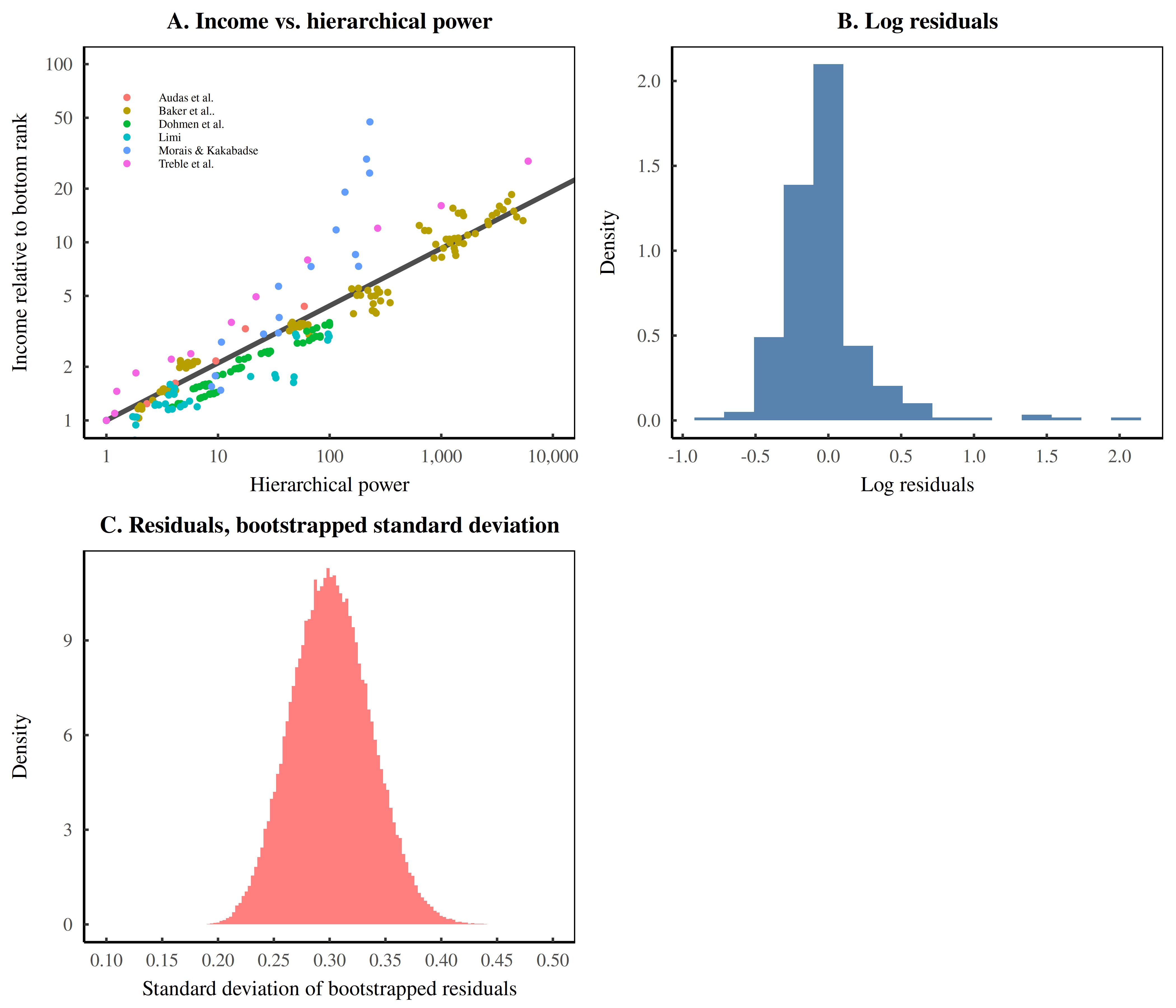Figure 18: Setting power-income noise. This figure illustrates how I model noise in the power-income relation. I start with data for case-study firms, shown in Panel A. I fit this data with a log-log regression, and then measure the log residuals, plotted in Panel B. To model these residuals, I bootstrap their standard deviation. Panel C shows the resulting distribution for the standard deviation. Each iteration of the hierarchy model samples from this distribution and uses the standard deviation to model power-income noise. Figure 18C shows the bootstrapped standard deviation of these residuals. (As a reminder, bootstrap statistics capture uncertainty by randomly sampling from the source data.) In this case, I randomly sample from the power-income residuals and then calculate their standard deviation. After many iterations, Figure 18C shows the resulting distribution. I use these bootstrapped standard deviations to model power-income noise. Each iteration of the hierarchy model takes a bootstrapped standard deviation, and inputs it into a lognormal distribution (as the shape parameter). I then generate power-income noise by drawing random numbers from this distribution. #### Hierarchy model My hierarchy model is based on equations derived independently by Herbert Simon and Harold Lydall. In this model, hierarchies have a constant span of control. We assume that there is one person in the top rank. The total membership in the hierarchy is then given by the following geometric series:  \displaystyle N_T = 1 + s +s^2 + \ldots + s^{n-1} (1) Here n is the number of ranks, s is the span of control, and N_T is the total membership. Summing this geometric series gives:  \displaystyle N_T = \frac{1-s^{n}}{1-s} (2) In this model, the input is the hierarchy size N_T and the span of control s . To model the hierarchy, we must first estimate the number of hierarchical ranks n . To do this, we solve the equation above for n , giving:  \displaystyle n = \left\lfloor~ \frac{\log \left[ 1 + N_T(s-1) \right]}{\log(s)} ~\right\rfloor (3) Here \lfloor\rfloor denotes rounding down to the nearest integer. Next we calculate N_1 — the employment in the bottom hierarchical rank. To do this, we first note that the firm’s total membership N_T is given by the following geometric series:  \displaystyle N_T = N_1 \left( 1 + \frac{1}{s} + \frac{1}{s^2} + \ldots + \frac{1}{s^{n-1}} \right) (4) Summing this series gives:  \displaystyle N_T = N_1 \left( \frac{1-1/s^{n}}{1-1/s} \right) (5) Solving for N_1 gives:  \displaystyle N_1 = N_T \left( \frac{1 - 1/s}{1-1/s^{n}} \right) (6) Given N_1 , membership in each hierarchical rank h is:  \displaystyle N_h = \left\lfloor \frac{N_1}{s^{h-1}} \right\rfloor (7) Sometimes rounding errors cause the total employment of the modeled hierarchy to depart slightly from the size of the original input value. When this happens I add/subtract members from the bottom rank to correct the error. Once the hierarchy has been constructed, I model income ( I ) as a function of hierarchical power:  \displaystyle I = N (\bar{P}_h)^D (8) Here D is the ‘degree of hierarchical despotism’ — a parameter that determines how rapidly income grows with hierarchical power. N is statistical noise generated by drawing random numbers from a lognormal distribution. \bar{P}_h is the average hierarchical power (per person) associated with rank h . It is defined as  \displaystyle \bar{P}_{h} = 1 + \bar{S}_h (9) where \bar{S}_h is the average number of subordinates per member of rank h :  \displaystyle \bar{S}_h ~ = \sum_{i = 1}^{h -1} \frac{N_i}{N_h} (10) The model is implemented numerically in C++, using the Armadillo linear algebra library. For R users, I have created R functions for the model, available at GitHub: #### Setting the hierarchy model’s fixed parameters The three parameters below are fixed by empirical data. To capture uncertainty in the underlying data, these parameters vary stochastically between model iterations. Importantly, however, these parameters have no time-based trend. Size distribution of firms. The hierarchy model starts with a size distribution of firms drawn from a discrete power-law distribution. I set the exponent of this power-law distribution by fitting a power law to US empirical data, as shown in Figure 16. Since this fitted power-law exponent has changed with time, the model samples randomly from the various fits, using a different value for each iteration. Span of control. The span of control is determined from the average span found in case-study data, as shown in Figure 17 for details. The average span is close to 3, but due to the small sample size, comes with significant uncertainty. To incorporate this uncertainty, each iteration of the model samples from the span of control distribution and uses the resulting bootstrapped mean. Power-income noise. I generate power-income noise using a lognormal distribution. Here’s how I set the shape parameter, \sigma . I start with the power-income relation found in case study firms, shown in Figure 18. After fitting a log-log trend to this data, I then calculate the log residuals. The scale parameter \sigma then equals the standard deviation of these log residuals. To incorporate uncertainty, each iteration of the model samples randomly from these residuals and uses the resulting bootstrapped standard deviation. #### Estimating the degree of hierarchical despotism in the United States My thesis is that growing income inequality is being driven by a rise in hierarchical despotism within firms. To test this hypothesis, I use the hierarchy model to infer the degree of despotism within US society. (The inference is plotted in Figure 13.) Figure 19 illustrates my fitting method. I start with US income threshold data reported by the World Inequality Database. These thresholds represent the lowest income that will get you into a corresponding income percentile. For example, in 1981 an income of$205,628 bought you membership in the top 1% — the 99th percentile.

When we study the distribution of income, we don’t care about the absolute value of this threshold. Instead, we care about its relative size. To fit the hierarchy model to US data, I measure income thresholds relative to the 99th percentile. Then I keep only the percentiles about P99. (The reason for this cut of is that the hierarchy model is mostly concerned with the behavior of top incomes.)

Given these empirical income thresholds, I then look at the corresponding thresholds in the hierarchy model and choose the degree of despotism that minimizes the error.

Figure 19 shows some example fits. In both panels, the horizontal axis shows income percentile, with tick-marks corresponding to the percentiles reported by the World Inequality Database. The vertical axis shows the corresponding income threshold in the United States (blue) and the hierarchy model (red). As expected, the fitted values for hierarchical despotism are not constant. For example, a value of D=0.62 fits the data well in 1981 (top panel). In 2013, a value of D=0.76 fits the data (bottom panel). When we apply this method to recent US history, we get the pattern shown in Figure 13.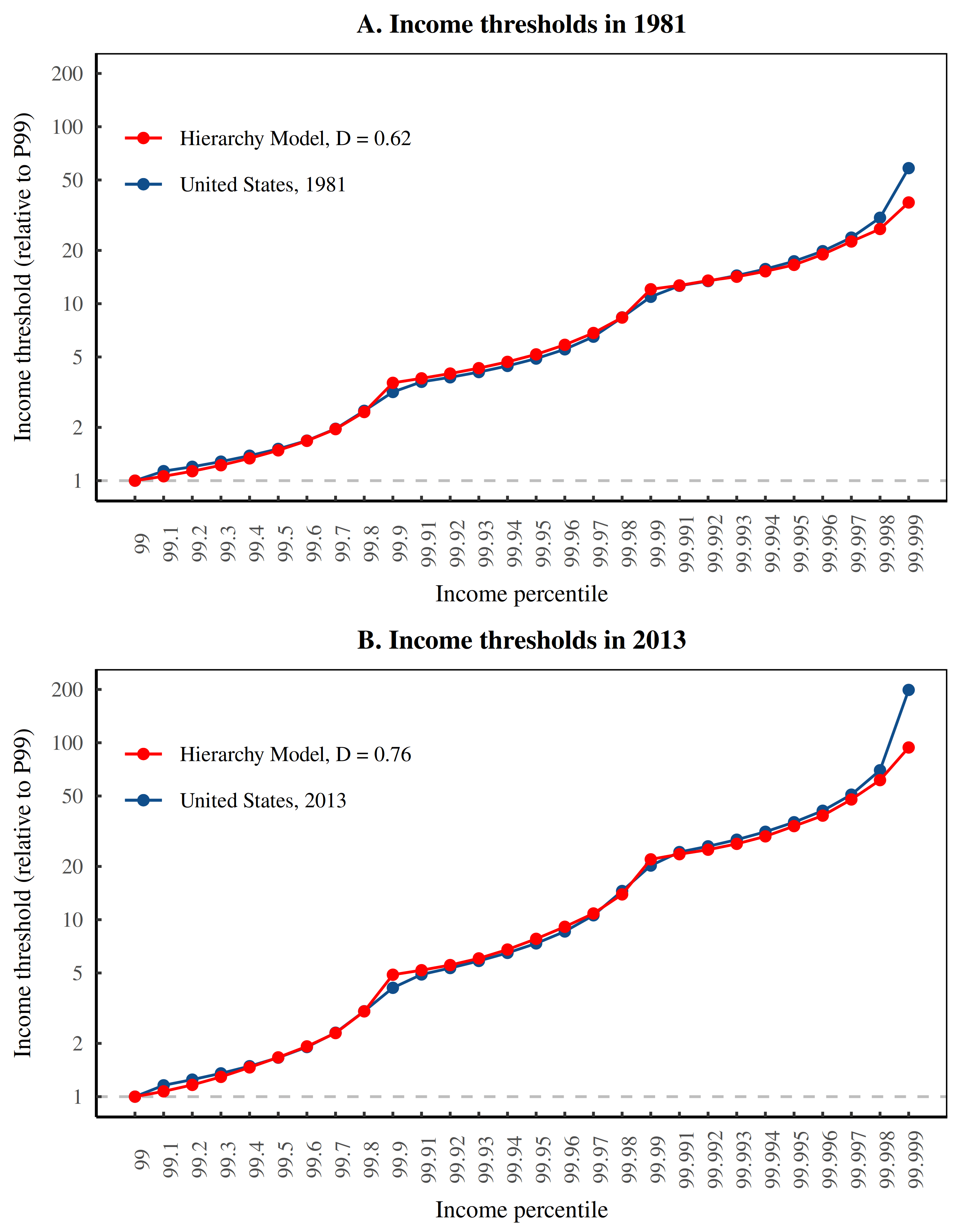Figure 19: Fitting the hierarchy model to US top incomes. This figure illustrates how I use the hierarchy model to estimate the degree of hierarchical despotism in the United States. I start by looking at income threshold data among the top 1%. In each panel the horizontal axis plots income percentile. (Note that the horizontal scale is categorical, giving equal distance between percentile gaps that are not actually equal.) The vertical axis shows the corresponding income threshold, normalized so that the P99 threshold is 1. Given the empirical thresholds (blue), I fit the hierarchy model to the data by minimizing the threshold error. The red curves show some example fits. As expected, the best-fit value of hierarchical despotism (D) varies with time. In 1981, it is roughly 0.62. In 2013, the best-fit value of D increased to 0.76.

### The tuning test

Another way to test the US hierarchy model is to tune it on different sets of data and see if the resulting parameters are the same. Figure 20 shows such a test. Here, I show three different estimates for how US hierarchical despotism changed from 1981 to 2013.

The test works as follows. I start with value of despotism in 1981 (D = 0.59), inferred by the hierarchy model using the distribution of top US incomes. Although this value has some uncertainty attached to it, let’s assume it is accurate. Next, I tune the hierarchy model to US data in 2013, and measure the change in despotism.

In Figure 20, the blue boxplot shows the change inferred from top US incomes (the values plotted in Figure 13). The green boxplot shows the change in despotism estimated by fitting Song’s within-firm data, restricted to the top 1% of incomes. (the data shown in Figure 15). As a reminder, this is counterfactual data that measures how income changed as a function of income percentile, assuming the average income within firms remained constant. Finally, the red boxplot shows the change in despotism inferred from fitting Song’s within-firm data among the bottom 99% (the data in Figure 14).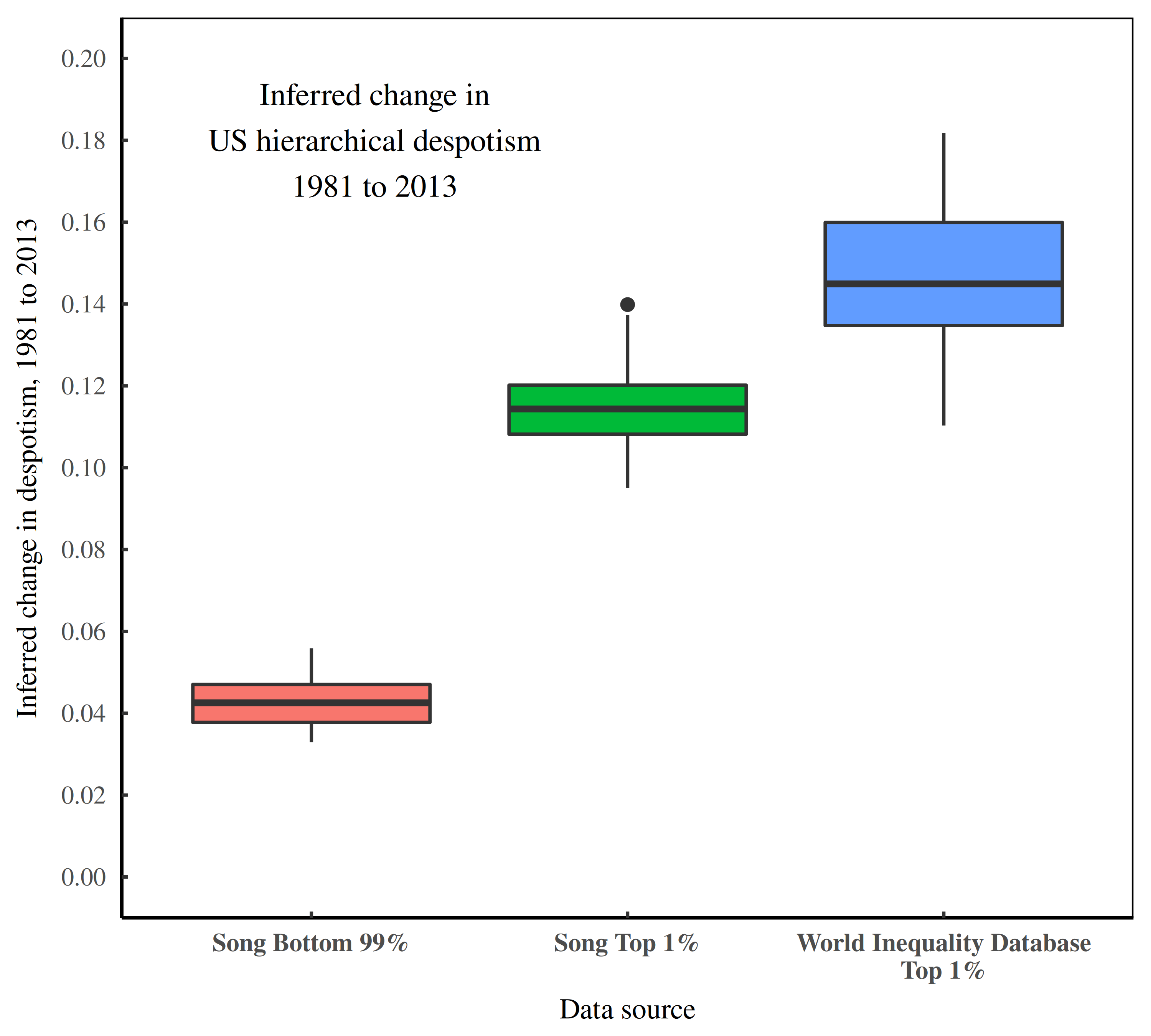Figure 20: The change in US hierarchical despotism from 1981 to 2013, inferred from three different sources. This figure shows three different estimates for the change in hierarchical despotism within US firms between 1981 and 2013. The red boxplot shows changes in hierarchical despotism that best fit Song’s estimates for within-firm income change among the bottom 99% (Fig. 14). The green boxplot shows the change in despotism that best fits Song’s within-firm redistribution among the top 1% (Fig. 15). The blue boxplot shows the change in despotism estimated from US top incomes (Fig. 13). Note: the change in despotism is measured relative to the 1981 value of D = 0.59, inferred from US top incomes. [Sources and methods].

Looking at Figure 20, the inferences based on top incomes are consistent with each other. In other words, independent estimates point to roughly the same change in hierarchical despotism between 1981 and 2013. That’s reassuring.

However, our estimate based on bottom incomes gives a different result. What’s going on? The short answer is that I don’t know. My hunch, though, is that power-income hypothesis breaks down among bottom incomes. Basically, I’ve looked at the relation between income and hierarchical power and supposed we can model it with straight line. Among individuals with significant hierarchical power, that seems like a good model. But perhaps at the bottom of hierarchy, the power-income relation is a bit more complicated. For now, we’ll leave the question open.

#### The span of the powerless

When we redistribute income by ramping up hierarchical despotism, the L-shaped pattern (shown in Figure 11) is unavoidable. Exactly where the L begins, though, depends on the shape of the hierarchy. And that shape, in turn, depends on the span of control.

In a large hierarchy with a span of control s, the portion of people in the bottom hierarchical rank is:

\displaystyle \text{portion in bottom rank} = \frac{s - 1}{s}

So if the span of control is 2, then 50% of people sit in the bottom hierarchical rank. Or if the span of control is 10, then 90% of people sit in the bottom rank. And so on.

When it comes to income, the span of control determines how many people are unaffected by the returns to hierarchical power. The larger the span of control, the more people who receive no income boost when despotism increases. Figure 21 demonstrates.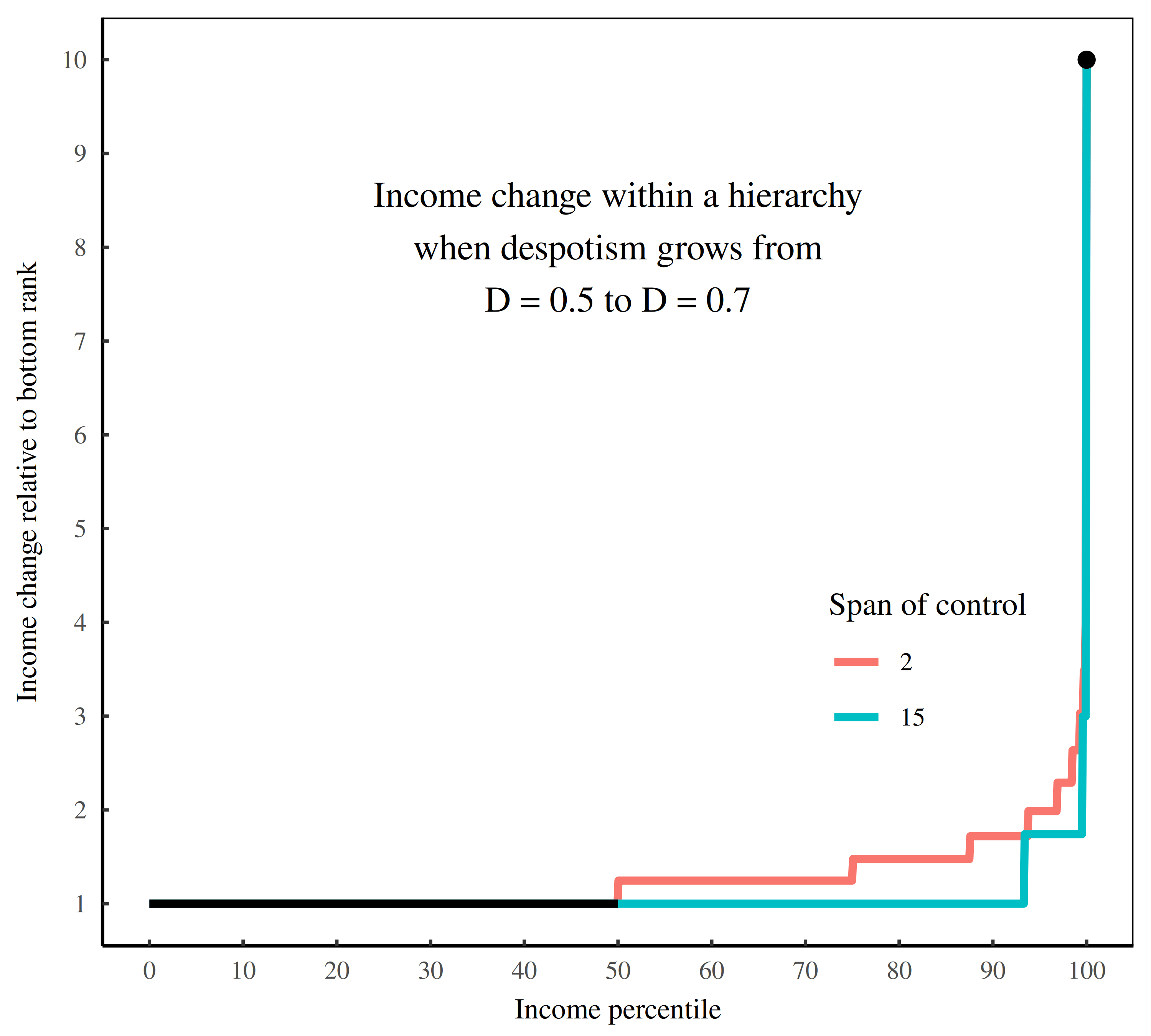Figure 21: How the span of control affects income redistribution. This figure continues the model of hierarchy illustrated in Figure 9 — one with 100K members. Here, I illustrate how the span of control affects income redistribution. The span determines how many people have no subordinates, and therefore, receive no income returns to hierarchical power. The larger the span, the more people in the bottom rank, and therefore the more people who’s income doesn’t change when we ramp up hierarchical despotism.
1. People sometimes point to the Catholic church as an example of a hierarchy in which the ruler receives the same income as his subordinates. Actually, the Catholic hierarchy seems to have an inverted pay grade: the pope apparently receives no salary at all. But this lack of salary is just an artifact of the Church’s feudal origins. Kings didn’t pay themselves salaries, yet they nonetheless commanded immense wealth. And so it goes with the pope, who lives in a gilded palace that most kings would envy.↩︎
2. In Figure 9, the ‘steps’ in hierarchical power are an artifact of my model, which assumes a constant span of control throughout the hierarchy. So everyone with the same rank has the same hierarchical power. In real-world hierarchies, we expect that the span of control will vary between individuals. As a result, there will be a ‘spread’ in hierarchical power within ranks, and a blurring of the step-like pattern. At least that’s my guess. To date, I’m not aware of any studies that have resolved the network structure of an entire chain of command.↩︎
3. In real-world hierarchies, there is admittedly no despotism dial — at least not in the simple sense modeled here. Corporate rulers do not prescribe income by counting subordinates and applying a scaling law. Nor do they choose to redistribute income by consciously altering this formula. As such, the fact that income tends to scale with hierarchical power is what scientists call an ‘emergent effect’. It is created by individual decisions that (unknowingly) add up to the effect we observe.

While we do not know these individual decisions, it seems likely they are governed by rules of thumb. For example, when you get promoted, it’s a universal norm that you should receive a pay raise. It is also a norm that this raise is multiplicative (rather than additive). In other words, each promotion bumps up your income a certain percentage (as opposed to a certain dollar amount). The effect of this norm is that income tends to grow exponentially with hierarchical rank. When you combine the exponential growth of income with the exponential growth of subordinates, you get a power-law relation between income and hierarchical power. (I review the math here.)

Although this pay-raise norm exists throughout the corporate hierarchy, it is constrained by the behavior at the top and the bottom. For example, if the CEO decides to give himself a raise, the effect will ripple down the hierarchy. Other execs will seek a raise that is slightly more modest, and this change will propagate down the chain of command. And so the hierarchy will become more despotic (as I’ve defined it).

Alternatively, bottom-ranked employees might unionize and fight for a raise. If they succeed (and the top brass keep their income the same), then the hierarchy’s pay grades will ‘flatten’. And so the hierarchy will become less despotic.

To summarize, the tidy statistic that I call ‘hierarchical despotism’ is likely the result of a messy power struggle within the corporate hierarchy.↩︎

4. Here’s what I mean by ‘iteration’ of the hierarchy model. Recall that the model generates a size distribution of firms by sampling random numbers from a power-law distribution. Because this sample changes each time we draw it, the results of the hierarchy model are not constant. They vary between iterations; each time we press RUN, we get something slightly different. The way we study this type of model is by running it many times, and then measuring the range of results. It would be a mess, however, if we tried plot this range as a landscape. That’s why Figure 12 visualizes a single iteration of the model.↩︎
5. Actually, it is possible for inequality to increase, but for the hierarchy model to not infer a change in despotism. For that to happen, the distribution of top incomes (i.e. the upper tail of the distribution) would have to remain unchanged. Instead, the bottom of the distribution would have to drop out. In effect, the poor would get poorer, but the rich would not get richer. (If you’re interested in the mathematics of this scenario, I discussed them here. I also looked for evidence here.)

While we can easily model the mathematics of this income-collapse scenario, it turns out to be uncommon in the real world. That’s because it assumes a collapse of the social safety net — something that is relatively rare. Yes, people may tolerate their neighbors getting richer. But despots aside, few people will live with plenty while their neighbors starve. As a result, changes in inequality are usually driven by the redistribution of top incomes. In short, when inequality increases, it’s typically because the rich get richer. As such, given increasing inequality, the hierarchy model is nearly guaranteed to infer growing hierarchy despotism.↩︎

6. The apparent rise or fall of income depends on where we ‘normalize’ the distribution — the place where we hold income constant. When we measure income relative to the firm mean, then ramping up despotism causes the income at the bottom of the hierarchy to drop slightly. That’s because as the rich get richer, the mean income rises.↩︎
7. If you think it odd to treat firms as units of power, I recommend you read Jonathan Nitzan and Shimshon Bichler’s book Capital as Power. They make the book-length case that the structure of corporations has little to do with ‘production’ and everything to do with power.↩︎

Fix, B. (2018). Hierarchy and the power-law income distribution tail. Journal of Computational Social Science, 1(2), 471–491.

Fix, B. (2020). How the rich are different: Hierarchical power as the basis of income size and class. Journal of Computational Social Science, 1–52.

Fix, B. (2021). Redistributing income through hierarchy. Real-World Economics Review, (98), 58–86.

Song, J., Price, D. J., Guvenen, F., Bloom, N., & Von Wachter, T. (2019). Firming up inequality. The Quarterly Journal of Economics, 134(1), 1–50.

1. […] Firming Up Hierarchy […]

2. […] with avowed fascists than with any kind of social democracy, let alone “the left”.Firming Up HierarchyBlair Fix [Economics from the Top Down, via Mike Norman Economics 11-19-2022] I think that the […]

3. […] Firming Up Hierarchy Economics from the Top Down. Interesting! […]

4.Wolf1 says:

Interesting work, but perhaps the missing ingredient is the offshoring of labor and manufacturing from the US in the past several decades – a negative – combined with remaining labor law and cost of living effects on the positive side.

5.eg says:

I wonder how the increase in outsourcing maps onto changes in the despotism imputed here — I mean both in terms of international outsourcing (adding large numbers of even lower paid foreign workers) and the kind where certain functions (janitorial comes to mind) are hived off in order to get cheaper labour inputs than management might be comfortable having on its internal books (never mind the psychological and social costs of having to justify even wider in-firm pay scales and/or encountering those you are exploiting in this way as colleagues day-to-day).